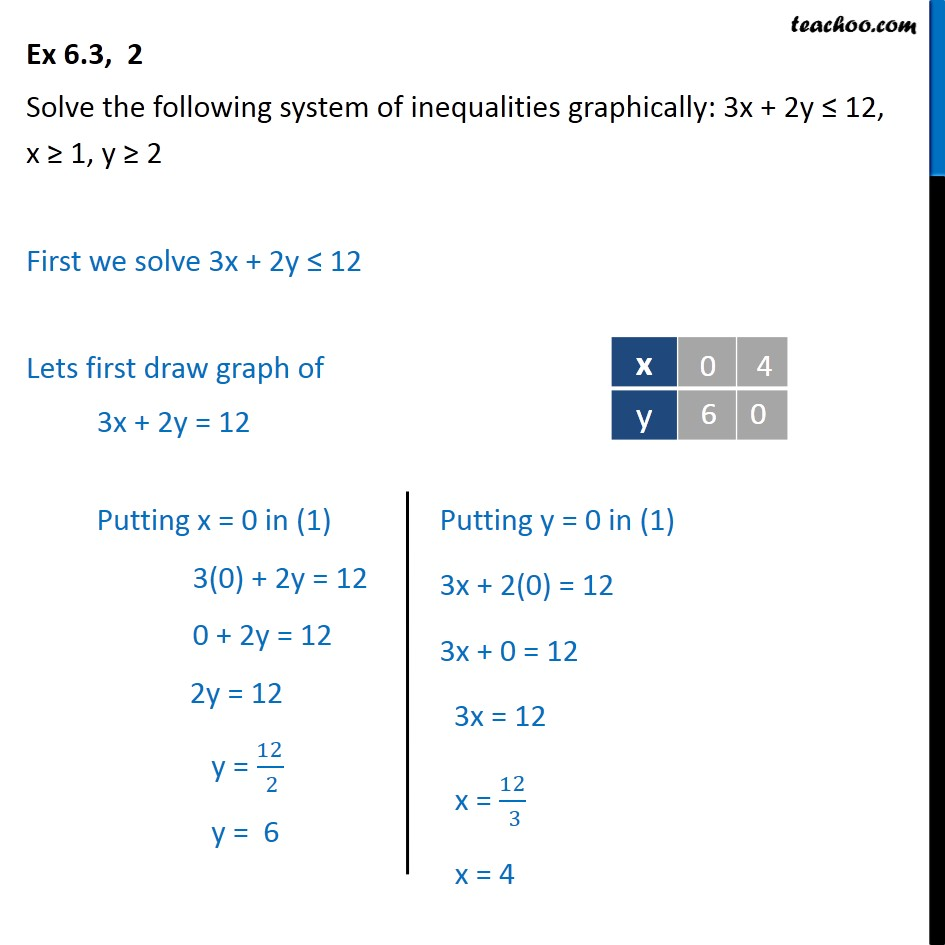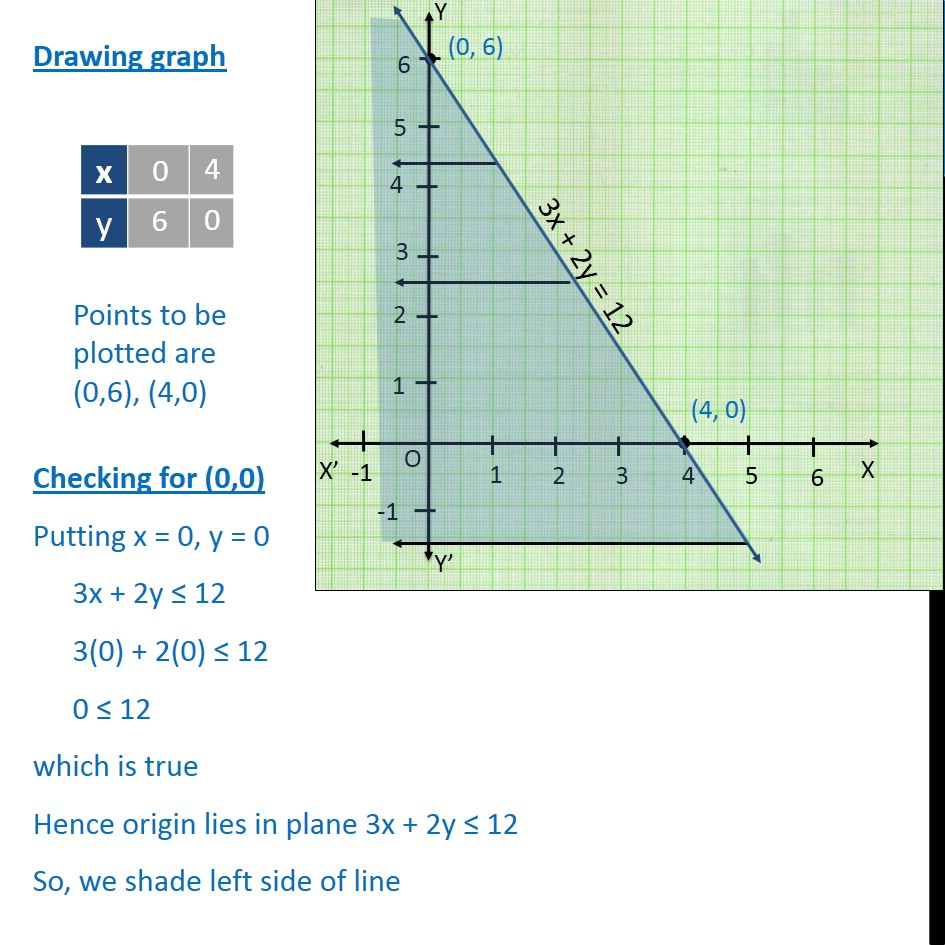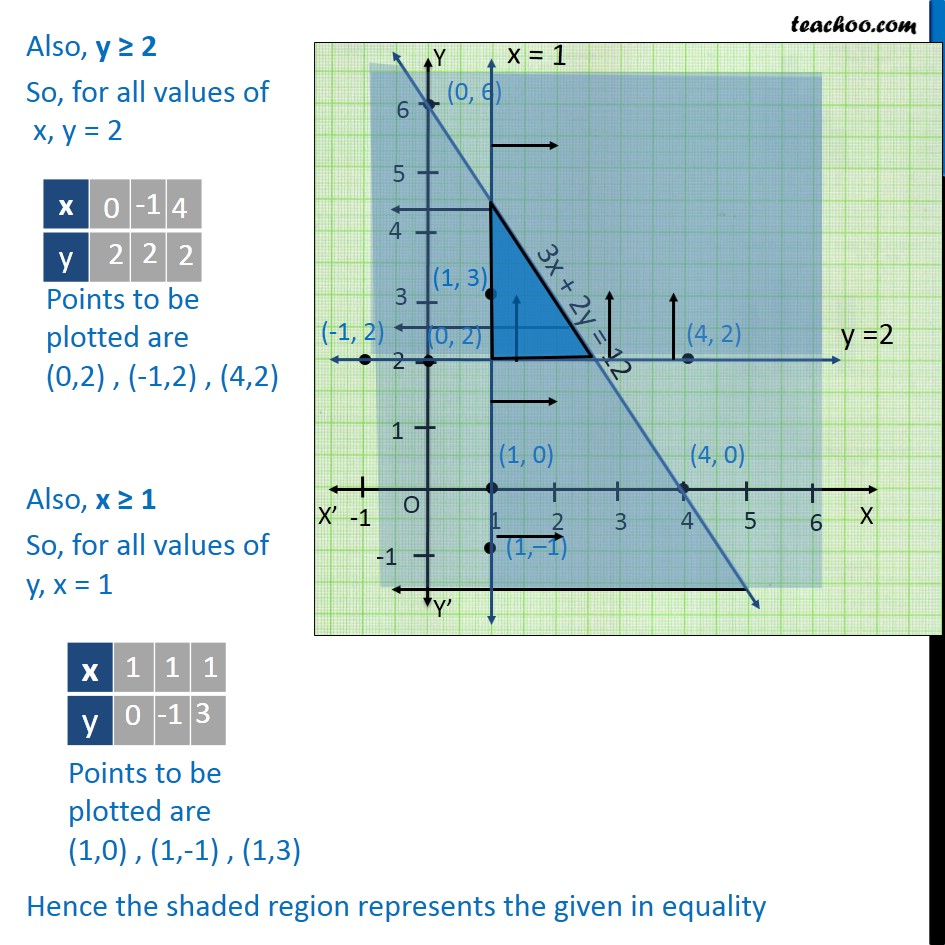1. Chapter 6 Class 11 Linear Inequalities
2. Serial order wise
3. Ex 6.3

Transcript

Ex6.3, 2 Solve the following system of inequalities graphically: 3x + 2y 12, x 1, y 2 First we solve 3x + 2y 12 Lets first draw graph of 3x + 2y = 12 Drawing graph Checking for (0,0) Putting x = 0, y = 0 3x + 2y 12 3(0) + 2(0) 12 0 12 which is true Hence origin lies in plane 3x + 2y 12 So, we shade left side of line Also, y 2 So, for all values of x, y = 2 Also, x 1 So, for all values of y, x = 1 Hence the shaded region represents the given in equality

Ex 6.3